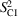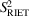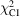International Tables for Crystallography (2019). Vol. H. ch. 4.3, pp. 414-432https://doi.org/10.1107/97809553602060000958

## Contents

• 4.3. Real-space methods for structure solution from powder-diffraction data: application to molecular structures  (pp. 414-432)
• 4.3.1. Introduction  (pp. 414-416) | html | pdf |
• 4.3.2. Real-space structure determination: a global-optimization extension of the Rietveld method  (p. 416) | html | pdf |
• 4.3.3. Optimizing the process of real-space structure determination from powder-diffraction data  (pp. 416-418) | html | pdf |
• 4.3.3.1. Introduction  (pp. 416-417) | html | pdf |
• 4.3.3.2. Parameterization of the molecular structure  (pp. 417-418) | html | pdf |
• 4.3.4. The nature and magnitude of the global-optimization challenge  (pp. 418-424) | html | pdf |
• 4.3.4.1. Interpretation of the least-squares cost function  (pp. 419-421) | html | pdf |
• 4.3.4.2. The chi-squared hypersurface  (pp. 421-424) | html | pdf |
• 4.3.5. Global-optimization algorithms  (pp. 424-430) | html | pdf |
• 4.3.5.1. Stochastic search algorithms  (pp. 425-429) | html | pdf |
• 4.3.5.1.1. Introduction  (p. 426) | html | pdf |
• 4.3.5.1.2. Monte Carlo methods  (p. 426) | html | pdf |
• 4.3.5.1.3. Simulated-annealing methods  (pp. 426-427) | html | pdf |
• 4.3.5.1.4. Parallel tempering  (pp. 427-428) | html | pdf |
• 4.3.5.1.5. Genetic algorithms  (pp. 428-429) | html | pdf |
• 4.3.5.1.6. Differential evolution  (p. 429) | html | pdf |
• 4.3.5.2. Deterministic search algorithms  (pp. 429-430) | html | pdf |
• 4.3.5.2.1. Introduction  (p. 429) | html | pdf |
• 4.3.5.2.2. The hybrid Monte Carlo algorithm  (pp. 429-430) | html | pdf |
• 4.3.6. Using maximum-likelihood techniques to tackle incomplete structural models  (pp. 430-431) | html | pdf |
• 4.3.6.1. Introduction  (p. 430) | html | pdf |
• 4.3.6.2. Working with incomplete structural models: maximum-likelihood methods  (pp. 430-431) | html | pdf |
• References | html | pdf |
• Figures
• Fig. 4.3.1. (a) The maze of strategies associated with the determination of crystal structures from powder-diffraction data (after Baerlocher & McCusker, 2002) and (b) the modified `global-optimization' maze showing the double start point and simplification of the principal maze  (p. 415) | html | pdf |
• Fig. 4.3.2. (a) The Cartesian molecular coordinate system for hydrochlorothiazide  (p. 417) | html | pdf |
• Fig. 4.3.3. The reported values for bothandin (a) the final stages of the structure-solution process for promazine hydrochloride  (p. 420) | html | pdf |
• Fig. 4.3.4. (a) The molecular structure and (b) the b-axis projection of the crystal structure of the polymorphic form B of famotidine  (p. 421) | html | pdf |
• Fig. 4.3.5. Thefrequency distribution for famotidine (form B)  (p. 421) | html | pdf |
• Fig. 4.3.6. Two-dimensional section of thehypersurface showing the variation inas function of x and z translations  (p. 422) | html | pdf |
• Fig. 4.3.7. Comparison of the crystal structure shifted by z = 1/4 with the correct crystal structure of famotidine (form B)  (p. 422) | html | pdf |
• Fig. 4.3.8. (a) The log-normal distribution of local minima obtained from steepest-descents minimization of 20 000 randomly selected famotidine form B crystal structures  (p. 423) | html | pdf |
• Fig. 4.3.9. Three good solutions for the crystal structure determination of famotidine form B  (p. 423) | html | pdf |
• Fig. 4.3.10. The crystal structure of famotidine form B associated with a Δx = 1/4 shift from the correct solution  (p. 424) | html | pdf |
• Fig. 4.3.11. The move-by-move change inobserved during a real-space structure-solution run for famotidine form B  (p. 424) | html | pdf |
• Fig. 4.3.12. (a) A simple periodic potential-energy function constructed over a square cell  (p. 425) | html | pdf |
• Fig. 4.3.13. Molecular structures of 1,4-bis(2-phenethyloxyethanesulfonyl)piperazine, famotidine, 2-{[3-(2-phenylethoxy)propyl]sulfonyl}ethyl benzoate and verapamil hydrochloride  (p. 426) | html | pdf |
• Fig. 4.3.14. The molecular structure and crystal structure of the malaria pigment B-haematin  (p. 427) | html | pdf |
• Fig. 4.3.15. A plot of the best χ2 values versus the number of χ2 evaluations for the 20 simulated-annealing structure-solution attempts for capsaicin (after Shankland et al  (p. 427) | html | pdf |
• Fig. 4.3.16. A single-point molecular crossover for cimetidine  (p. 428) | html | pdf |
• Fig. 4.3.17. Mutation of a single gene influencing the orientation of a cimetidine molecule within the unit cell  (p. 428) | html | pdf |
• Fig. 4.3.18. A plot of the best χ2 values versus the number of χ2 evaluations for the 20 hybrid Monte Carlo structure-solution attempts for capsaicin (after Shankland et al  (p. 429) | html | pdf |
• Fig. 4.3.19. (a) The correct crystal structure of remacemide nitrate  (p. 430) | html | pdf |
• Tables
• Table 4.3.1. The symbolic representation of the initial bonds, angles and dihedral angles described in the Z-matrix of hydrochlorothiazide  (p. 418) | html | pdf |
• Table 4.3.2. Z-matrix for hydrochlorothiazide  (p. 418) | html | pdf |
• Table 4.3.3. Estimated minimum d-spacing range extent (in Å) for structure solution for different complexities of molecular structures using low (5 × 10−3), medium (2 × 10−3) and high (2 × 10−4) resolution instrumentation  (p. 421) | html | pdf |